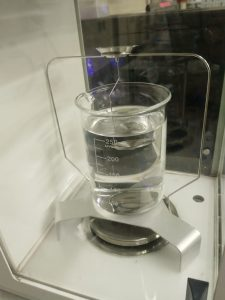*All of the information for this post was gathered from the sources listed below. Please use them for further reading on the subject.

The physical properties of a polymeric sample, such as density, ductility, and yeild strength, are all highly dependent on the amount of crystalline material (often called percent crystallinity) in the sample. There are two principal methods by which we are able to determine the percent crystallinity of a sample in the MCL: density measurements, and differential scanning calorimetry (DSC). Both of these methods compare the unknown sample to a fully crystalline or amorphous sample of the same polymer, and require the user to have some reference data. Additionally, DSC is a destructive technique, meaning that the provided sample should be one which is not necessary for further testing.

The most common method for determining the percent crystallinity of a sample involves comparing the density of the sample to the fully crystalline and fully amorphous densities. The MCL has an Archimedes Density Measurement tool which can be used in conjunction with a regular scale in order to determine the density of a sample. The figure below shows the proper setup for the attachment.In order to measure the density using this apparatus, first place your sample on the top basket and record the value. While the sample is still on the top basket, tare the scale and then move the sample to the bottom basket such that the sample is fully submerged in water and record that value. Finally, use a thermometer to determine the temperature of the water so you can find an accurate value for its density. To calculate the density of your sample, take the first value (dry mass), divide by the second value (buoyancy) and multiply the result by the density of the water. It is important to note that  this process does not work if your sample floats or dissolves in water. In that case, a different liquid could be used. Once the density of your sample has been determined, use the equation below to find the weight fraction crystallinity of your sample.

• χ is the weight fraction of crystalline material in your sample
• ρ is the total density of your sample
• ρa is the density of the fully amorphous polymer
• ρc is the density of the fully crystalline polymer

It is also possible to determine the percent crystallinity of a polymer using DSC measurements. In this case, you will need to know the specific heat capacities of purely crystalline and purely amorphous samples of the polymer in order to complete the calculations. It is also helpful to know approximately what the melting temperature of your sample will be. In order to find percent crystallinity, run a DSC scan of the sample from room temperature to a temperature above the melting point. The result of this scan will be a curve with a few humps or peaks. There are three main pieces of data from this curve. The first (ΔH12) will the the integral across the whole temperature range, from the starting to the ending temperature. The second (ΔHf,m) will be the enthalpy of fusion at the melting point, determined by the area under the melting peak. The third (Tm) will be the melting point temperature, usually reported as the onset of the melting peak.

Along with these three pieces of data, you will also need to perform a few calculations. First, find ΔH21 using the amorphous sample’s specific heat capacity.

Next, find the enthalpy of fusion at room temperature using the enthalpy of fusion at the melting point, the difference between the amorphous and crystalline specific heat capacities, and the melting temperature.

Once those values are calculated, they can be plugged into a final equation in order to determine the percent crystallinity by weight at room temperature.